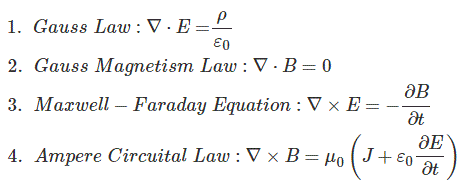# Electromagnetic Theory

Electromagnetism is the phenomenon which deals with the interaction between an Electric field and a magnetic Field. Stationary charges in a system lead to an electric field and moving charges in a system lead to a magnetic field. The direction of electric field and Magnetic field is always perpendicular to each other, and the wave travels at the speed of light. The Scottish scientist and mathematician James Clerk Maxwell, brought the correlation between electricity and magnetism for the first time using Maxwell’s equations. Maxwell’s four (4) differential equations unified existing laws of electricity and magnetism such as Newton’s
Law, Faraday’s Law, Kelvin’s law and Ampere Law.

Before learning Maxwell’s Equations, we need to learn 3 Mathematical operations which are basic entities of the equations. The Del operator refers to the partial differentiation of a function. We represent it as ∇ (Nabla). Grad f gives the gradient of a function i.e. grad f = ∇f, which means the partial differentiation of a function with respect to x, y and z-axis in a 3 – dimensional domain. The gradient is a vector quantity. The Divergence operator of a vector quantity gives us a scalar entity, which represents the rate at which the density exits a given range of space. It is represented as div v = ∇.v. The Curl represents the rotation of a vector in a three-dimensional field. It is denoted by Curl v = ∇ x v.
The 4 basic Maxwell’s Equations are as follows:-Here, ρ represents net charge inside the surface, ε0 represents permittivity of vacuum, B represents the magnetic field, E represents electric field and J represents current density. The first law states that the electric flux which forms across a closed surface is proportional to the charge enclosed. The second law states that the magnetic flux induced across a closed surface is zero. The third law states that the magnetic fields which vary with time lead to an electric field. The fourth law states that that time-varying Electric fields or steady currents lead to a magnetic field.

Hence, as shown by the above equations, it is proved that a varying electric field leads to a magnetic field and a varying Magnetic field leads to an electric field. The solution of Maxwell’s equations is a three-dimensional equation which represents a wave travelling at the speed of light. The electromagnetic energy waves carry energy through empty space and this energy is used for a variety of applications such as Remote sensing techniques, Radio waves, Ultraviolet (UV) rays and many more.

Want To Learn Faster? 🎓
Get electrical articles delivered to your inbox every week.
No credit card required—it’s 100% free.